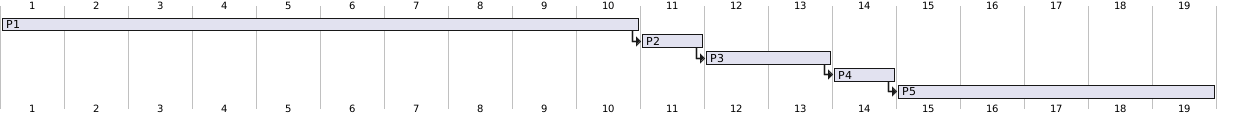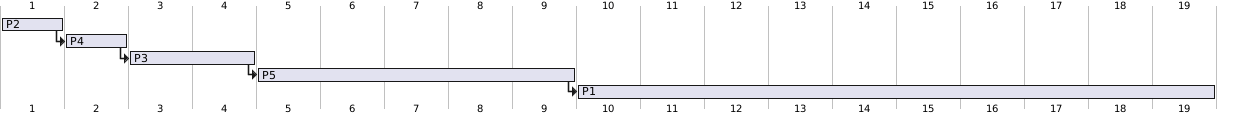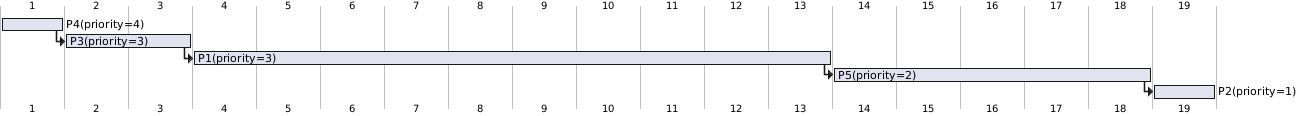5.1 Why is it important for the scheduler to distinguish I/O-bound programs from CPU-bound programs?

5.2 Discuss how the following pairs of scheduling criteria conlict in certain settings.

a. CPU utilization and response time b. Average turnaround time and maximum waiting time c. I/O device utilization and CPU utilization

a. CPU 利用率高，则要减小上下文切换次数，在极端假设下，必须一个进程执行完，再执行另一个进程，响应时间会变慢。

b. 平均轮转时间越小，则需要先执行区间小的程序，则存在一些程序优先级一直很低，产生饥荒现象。

c. I/O 操作对 CPU 利用不高，但是需要和设备交互，所以对上下文切换的要求高，导致 CPU 利用率下降。

5.3 Consider the exponential average formula used to predict the length ofthe next CPU burst. What are the implications of assigning the following values to the parameters used by the algorithm?

a. $\alpha = 0$ and $\tau _ 0 = 100 \text{ms}$

b. $\alpha = 0.99$ and $\tau _ 0 = 10 \text{ms}$

5.4 Consider the following set of processes, with the length of the CPU -bursttime given in milliseconds:

$$\begin{array}{ccc} Process & { Burst Time } & { Priority } \\ \hline P_{1} & 10 & 3 \\ P_{2} & 1 & 1 \\ P_{3} & 2 & 3 \\ P_{4} & 1 & 4 \\ P_{5} & 5 & 2 \end{array}$$

The processes are assumed to have arrived in the order $P_{1}, P_{2}, P_{3}, P_{4}, P_{5}$, all at time 0 .

a. Draw four Gantt charts illustrating the execution of these processes using FCFS , SJF , a nonpreemptive priority (a smaller priority number implies a higher priority), and RR (quantum = 1)scheduling.

FCFS 调度，先来先办：

@startgantt
projectscale daily zoom 4
[P1] lasts 10 days
[P2] lasts 1  days
[P2] starts at [P1]'s end
[P3] lasts 2  days
[P3] starts at [P2]'s end
[P4] lasts 1  days
[P4] starts at [P3]'s end
[P5] lasts 5  days
[P5] starts at [P4]'s end
@endganttSJF 调度，最短优先：

@startgantt
projectscale daily zoom 4
[P2] lasts 1  days
[P4] lasts 1  days
[P4] starts at [P2]'s end
[P3] lasts 2  days
[P3] starts at [P4]'s end
[P5] lasts 5  days
[P5] starts at [P3]'s end
[P1] lasts 10 days
[P1] starts at [P5]'s end
@endganttnonpreemptive priority，非抢占式，优先值越低优先值越高：

@startgantt
projectscale daily zoom 4
[P4(priority=4)] lasts 1  days
[P3(priority=3)] lasts 2  days
[P3(priority=3)] starts at [P4(priority=4)]'s end
[P1(priority=3)] lasts 10 days
[P1(priority=3)] starts at [P3(priority=3)]'s end
[P5(priority=2)] lasts 5  days
[P5(priority=2)] starts at [P1(priority=3)]'s end
[P2(priority=1)] lasts 1  days
[P2(priority=1)] starts at [P5(priority=2)]'s end
@endganttRR 轮转抢占式调度b. What is the turnaround time of each process for each of thescheduling algorithms in part a?

Pn FCFS SJF Pr RR
P1 10 10 13 19
P2 11 1 19 2
P3 13 4 3 7
P4 14 2 1 4
P5 19 9 18 14

c. What is the waiting time of each process for each of the schedulingalgorithms in part a?

Pn FCFS SJF Pr RR
P1 0 9 3 9
P2 10 0 18 1
P3 11 2 1 7
P4 13 1 0 5
P5 14 4 13 9

d. Which of the schedules in part a results in the minimal average waiting time (over all processes)?

5.5 Which of the following scheduling algorithms could result in starvation?

a. First-come, first-served

b. Shortest job irst

c. Round robin

d. Priority

5.6 Consider a variant of the RR scheduling algorithm where the entries in the ready queue are pointers to the PCBs.

a. What would be the effect of putting two pointers to the same process in the ready queue?

b. What would be the major advantages and disadvantages of this scheme?

c. How would you modify the basic RR algorithm to achieve the same effect without the duplicate pointers?

5.7 Consider a system running ten I/O-bound tasks and one CPU-bound task.

Assume that the I/O-bound tasks issue an I/O operation once for every millisecond of CPU computing and that each I/O operation takes 10 milliseconds to complete.

Also assume that the context switching overhead is 0.1 millisecond and that all processes are long-running tasks. What is the CPU utilization for a round-robin scheduler when:

a. The time quantum is 1 millisecond

b. The time quantum is 10 milliseconds

a 则产生一个 IO 请求并切换上下文的时间是 1.1ms，10 个耗时 11ms，而计算密集任务需要 10ms + 切换 10 次上下文 = 11ms，一共 22ms，而实际使用时间 1*10+10=20，利用率 20/22=91%

b. 则产生一个 IO 请求并切换上下文的时间是 1.1ms，10 个耗时 11ms，计算密集任务 + 切换上下文耗时 10.1ms，一共是 21.1ms，而实际使用时间 1*10+10=20，利用率 20/21.1=94%

5.8 Consider a system implementing multilevel queue scheduling. What strategy can a computer user employ to maximize the amount of CPU time allocated to the user’s process?

• 规则 3：工作进入系统时，放在最高优先级（最上层队列）。
• 规则 4a：工作用完整个时间片后，降低其优先级（移入下一个队列）。
• 规则 4b：如果工作在其时间片以内主动释放 CPU，则优先级不变。

5.9 Consider a preemptive priority scheduling algorithm based on dynami-cally changing priorities. Larger priority numbers imply higher priority.When a process is waiting for the CPU (in the ready queue, but not run-ning), its priority changes at a rate $\alpha$; when it is running, its prioritychanges at a rate $\beta$. All processes are given a priority of 0 when theyenter the ready queue. The parameters $\alpha$; and $\beta$ can be set to give many different scheduling algorithms.

a. What is the algorithm that results from $\beta > \alpha > 0$

FCFS

b. What is the algorithm that results from $\alpha < \beta < 0$ >

LIFO

5.10 Explain the differences in the degree to which the following scheduling algorithms discriminate in favor of short processes:

a. FCFS

b. RR

c. MLFQ

5.11 Using the Windows XP scheduling algorithm, what is the numeric priority of a thread for the following scenarios?

a. A thread in the REALTIME PRIORITY CLASS with a relative priority of HIGHEST.

b. A thread in the NORMAL PRIORITY CLASS with a relative priority of NORMAL.

c. A thread in the HIGH PRIORITY CLASS with a relative priority of ABOVE NORMAL.a. 26, b.8, c. 14

5.12 Consider the scheduling algorithm in the Solaris operating system fortime sharing threads:a. What is the time quantum (in milliseconds) for a thread with priority 10? With priority 55?

160,40

b. Assume a thread with priority 35 has used its entire time quantumwithout blocking. What new priority will the scheduler assign thisthread?

35

c. Assume a thread with priority 35 blocks for I/O before its timequantum has expired. What new priority will the scheduler assignthis thread?

54

5.13 The traditional UNIX scheduler enforces an inverse relationship between priority numbers and priorities: The higher the number, the lower the priority. The scheduler recalculates process priorities once per second using the following function:

$$\text { Priority }=(\text { Recent } \text { CPU usage } / 2)+\text { Base }$$

where base $=60$ and recent CPU usage refers to a value indicating how often a process has used the CPU since priorities were last recalculated. Assume that recent CPU usage for process $P_{1}$ is 40, process $P_{2}$ is 18 , and process $P_{3}$ is $10 .$ What will be the new priorities for these three processes when priorities are recalculated? Based on this information, does the traditional UNIX scheduler raise or lower the relative priority of a CPU-bound process?